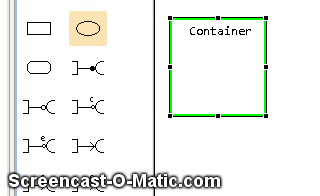# Tag: Donald Duck

Previous tutorial: Creating an OPM GEF Editor – Part 23: Drag & Drop from the Palette

Giving feedback to the user is very important in graphical editors which may have lots of information and we would like to minimize the amount of mistakes the user does. One example of feedback is coloring a figure when it is the target of an “add” operations – for example, color the Object to which a State is being added. The user can see very fast the change in the object and act upon the change (validate/cancel).

This is one of the places where the investment in GEF gives its fruits. Adding feedback seemed so simple that I thought is wouldn’t work. Feedback is handled by the `EditPolicy` in charge of creating new model elements, in my case, `OPMContainerXYLayoutPolicy`. Two functions were overriden to provide feedback: `showLayoutTargetFeedback` and `eraseLayoutTargetFeedback`. Both of these functions are called automatically by the GEF framework. To show how this works, I decided to paint the target figure a light blue color and to add a Donald Duck icon that moves with the cursor while you can apply the policy. This is the code that implements this functionality:

```  @Override
protected void showLayoutTargetFeedback(Request request) {
if(getHostFigure() instanceof OPMThingFigure) {
OPMThingFigure figure = (OPMThingFigure) getHostFigure();
figure.setBackgroundColor(ColorConstants.lightBlue);
figure.setOpaque(true);
// Adding a new ellipse somewhere in the screen:
if(!request.getExtendedData().containsKey("feedbackFigure")) {
IFigure feedbackFigure =
new ImageFigure(ImageDescriptor.createFromFile(this.getClass(), "../icons/dd.png").createImage());
feedbackFigure.setLocation(((DropRequest) request).getLocation());
feedbackFigure.setSize(feedbackFigure.getPreferredSize());
request.getExtendedData().put("feedbackFigure", feedbackFigure);
} else {
IFigure feedbackFigure = (IFigure) request.getExtendedData().remove("feedbackFigure");
feedbackFigure.setLocation(((DropRequest) request).getLocation());
request.getExtendedData().put("feedbackFigure", feedbackFigure);
}
}
}

@Override
protected void eraseLayoutTargetFeedback(Request request) {
if(getHostFigure() instanceof OPMThingFigure) {
OPMThingFigure figure = (OPMThingFigure) getHostFigure();
figure.setBackgroundColor(ColorConstants.white);
figure.setOpaque(false);
IFigure feedbackFigure = (IFigure) request.getExtendedData().remove("feedbackFigure");
removeFeedback(feedbackFigure);
}
}
```

And this is how it looks (thanks to Screencast-O-Matic):Cool, Ah? As usual, the source code for this example (as part of the whole project) can be found here.